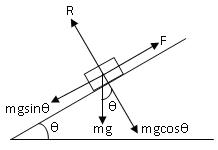Click to Chat

1800-1023-196

+91-120-4616500

CART 0

• 0

MY CART (5)

Use Coupon: CART20 and get 20% off on all online Study Material

ITEM
DETAILS
MRP
DISCOUNT
FINAL PRICE
Total Price: Rs.

There are no items in this cart.
Continue Shopping
`        Derive relation between angle of friction and angle of repose `
one year ago

```							Dear MaheshSuppose angle of friction = alpha and angle of repose = theta Let us suppose a body is placed on an inclined plane as in the above figure.Various forces involved are:- 1. weight,mg of the body,acting vertically downwards.2.normal reaction,R,acting perpendicular to inclined plane.3.Force of friction,F, acting up the plane.Now, mg can be resolved in two components:- mgcos theta opposite to Randmgsin theta opposite to FIn equilibrium, F = mgsin theta -------- eq.1R = mgcos theta ---------eq.2 On dividing eq.1 by eq.2we get, F/R = mgsin theta/mgcos thetamu = tan theta Where mu = coefficient of limiting friction.This is equal to the tangent of the angle of repose between two surfaces in contact. Now, since mu = tan alpha i.e coefficient of limiting friction between any two surfaces in contact is equal to tangent of the angle of friction between them.Thus, Theta = alpha i.e angle of friction is equal to angle of repose.
```
one year ago
Think You Can Provide A Better Answer ?

## Other Related Questions on 9 grade science

View all Questions »### Course Features

• 728 Video Lectures
• Revision Notes
• Previous Year Papers
• Mind Map
• Study Planner
• NCERT Solutions
• Discussion Forum
• Test paper with Video Solution### Course Features

• 731 Video Lectures
• Revision Notes
• Test paper with Video Solution
• Mind Map
• Study Planner
• NCERT Solutions
• Discussion Forum
• Previous Year Exam Questions